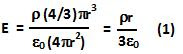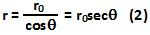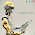## Sunday, March 28, 2010

### Irodov Problem 3.21The electric field at a distance r from the center of the sphere can be calculated using Gauss law easily as,E will be directed along the radius vector.

From elementary geometry we can see that,Hence,The component of electric field along the z-axis is given by,Equation (4) basically tells us that the component of electric field along the z-axis does not depend onin other words its same all over the disc section at a distance ro from the center.

The total area of the disc section is given by,Since the area vector of the disc section is directed along the z-axis, the total flux through this disc section is given by,1.This comment has been removed by the author.

2.all the solutions are great and have used good amount of calculus . by the way thanks , this is really helping me .

3.why can`t we take our gaussian surface as sphere.. won`t that be much easier

1.E.f is not uniform so gauss approach will no wrk

2.We don't take a sphere because we are asked to find the flux through a plane across the balls section.

4.how r°/r = cos theta

1.That's what I was wondering.

2.r is labelled incorrectly it is the radius of the dotted sphere I assume

5.That's what I was wondering.